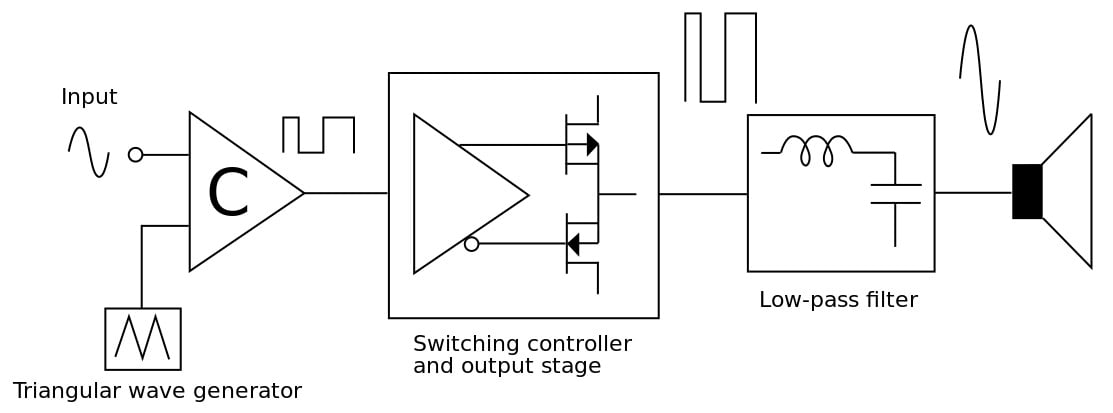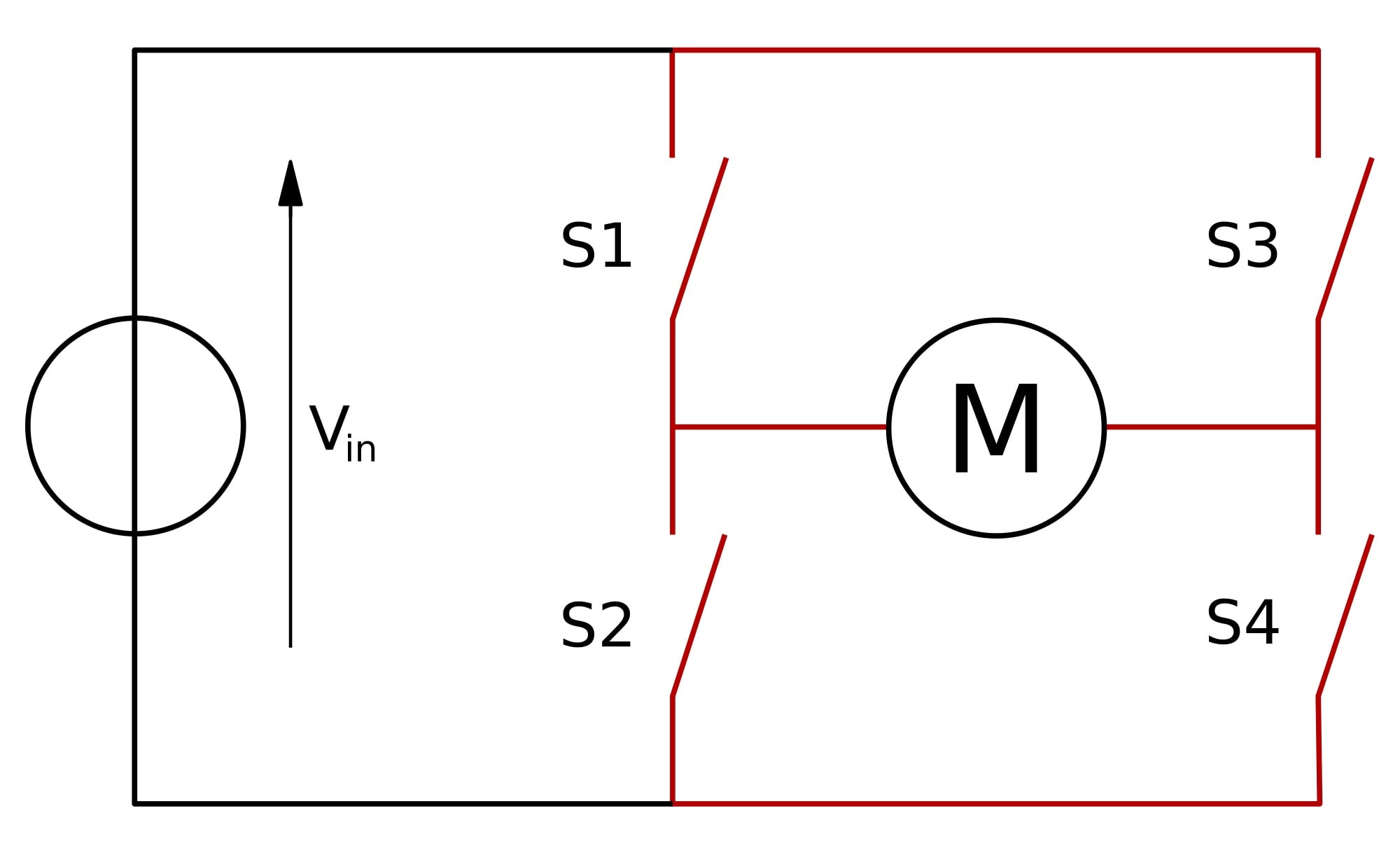Technical Article

# A Breakdown of Class D Amplifiers

January 21, 2016 by Ujjwal Roy

## Ever wanted to find out more about Class D amplification? Here's a handy breakdown.

### What's a Class D Amplifier?

A Class D Amplifier is basically a switching amplifier, or PWM amplifier. Compared to Class A, AB, and B amplifiers, the output-stage power dissipation in a Class D Amplifier is much lower, allowing the efficiency of these amplifiers to go above 90%. This difference gives the Class D significant advantages in portable audio solutions because the lower power dissipation produces less heat, saves circuit board space and, extends the battery life.

A Class D Amplifier has the following functional blocks:

### Input Filter

The input filter allows the amplifier to bias the input signal to the proper DC level for optimum operation. The input filter is a high pass filter that eliminates the DC component from the input signal. Input filter impacts the Low Frequency Pole and the gain and the power of the output signal.### Integrator

The integrator performs the mathematical operation of summing the input voltage and the feedback voltage. Integrating amplifier circuits impacts the low frequency pole and the bandwidth of the output.

### Reference Generator

The reference generator circuit is a voltage divider circuit that generates two reference voltages to power two comparator op-amps in the h-bridge control circuit. It also produces a common mode voltage which drives the integrator circuit. The closer the values of two reference voltages, the better the total harmonic distortion (THD) is.

### Power–on–Reset

The power-on-reset circuit provides an internal reset to all circuitry during initial power-up. It also monitors the power supplies to the IC, and it mutes the outputs and issues a reset when the voltages are lower than the minimum operating range. The power-on-reset circuit is responsible for supplying the power during the time it takes the level shifter to shift up to the required voltage.

### H-Bridge

The H-Bridge consists of a pair of PMOS and a pair of NMOS. The gates of these four transistors are driven by the four outputs from the H-Bridge controller. Full H-bridge circuits generally run from a single supply (VDD), with ground used for the negative supply terminal (VSS). For a given VDD and VSS, the differential nature of the bridge means that it can deliver twice the output signal and four times the output power of single-ended implementations. Only two sets of transistors out of four are on at a time. This lowers the power consumption and contributes to the efficiency of the amplifier.

#### Shoot Through Current in H-Bridge

A problem called “shoot through” can reduce the efficiency of class-D amplifiers and lead to potential operational failure. This occurs during the transition when one device is being cut off and another is being turned on. During the transition, both devices are on for a very small amount of time and a large current pulse can flow through the two. This can be eliminated by driving the gates of the MOSFETs with asymmetrical square waves using two comparators such that one device is cut off before the other is turned on.

#### Power Losses in H-Bridge

An important aspect in the design of MOSFET-based bridges is the size of the MOSFET. Optimum die size for minimal power loss depends on load impedance, required output power and the clock frequency. The bigger the size of the transistor die, the bigger the switching and gate loss. Bigger size reduces the conduction loss. MOSFET conduction losses are related to RDS(on), the Drain-Source resistance. RDS(on) is temperature-dependent, increasing when Temperature of Junction (TJ) increases. During the amplifier operation, Drain current determines the conduction losses as shown in the equation below:

P(conduction) = (ID RMS)2•RDS(on)

Amplifier efficiency depends on the MOSFET total power losses. The power loss in MOSFETs is the result of conduction, switching, and gate driven charge losses shown by the relation in equation given below:

Total Power Loss = P (switching)+P(conduction)

To minimize the switching loss and distortion, a power-on-reset circuit is used. The power-on-reset goes high if the level shifter’s capacitor is not properly charged and vice versa.

Moreover, the MOSFET’s power losses affect the MOSFET’s junction temperature TJ, as most of the power lost is converted into the heat. The junction temperature is an important design constraint as it determines the size of heatsink to be used.  High power loss increase TJ, and therefore, heatsink size.### H–Bridge Control

The H-bridge control controls the input voltages to be applied across the H-Bridge. The H-bridge has two comparators: D flip flops and two half-bridge switching circuits that supply pulses of opposite polarity to the MOSFETs. Two comparators are powered by the reference voltages from the reference generators. These produce a square wave output which is supplied to D-Flip-Flop as input. D-Flip-Flops act as a latch for the comparator output to sync it with a single clock that is fed into it. Buffers prevents the level shifter to discharge back into the H-Bridge control circuit.

### Level Shifter

The level shifter drives the PMOS gate voltage. The power-on-reset circuit is responsible for supplying the voltage during the time it takes the level shifter to shift up to the required voltage. It is important that the level shifter’s input capacitance is small to minimize the gate capacitance of the PMOS. The MOSFET gate capacitance should be small to minimize power dissipation and heating in the level shifter driving the MOSFET.

### Feedback Filter

The feedback filter circuit is a proportional feedback system. Feedback filters are used because high loop gain improves performance—suppressing distortion caused by nonlinearities in the forward path and reducing power supply noise by increasing the power-supply rejection (PSR).  The feedback voltage is proportional to the difference voltage of the left and right node voltages in H-Bridge.

### Clock Generator

The clock generator circuit produces a timing signal (known as a clock signal and behaves as such) that is used in synchronizing a circuit's operation. This circuit generates a square wave signal from 0V-5V. The frequency of this square wave signal acts as the sampling frequency for the input signal. The higher the sampling frequency, the lower the distortion in the output signal.